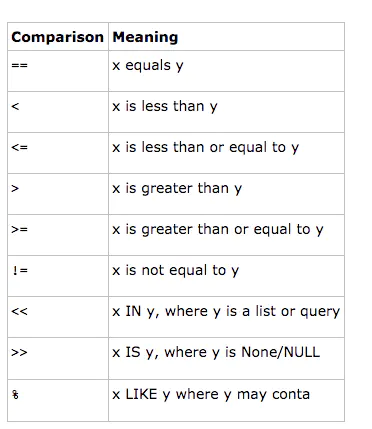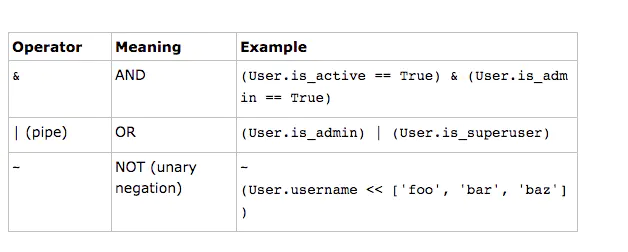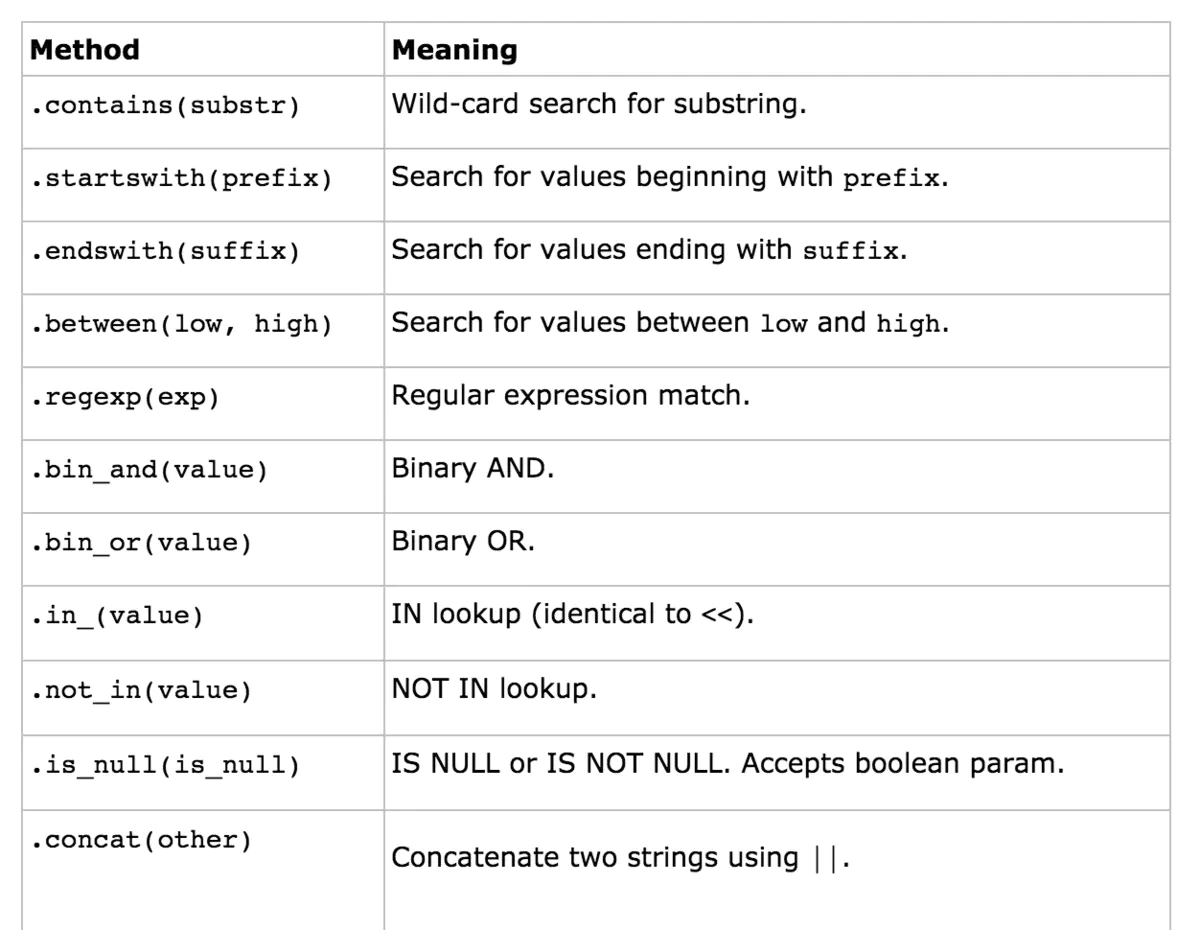﻿ bgods - [转] Peewee 使用（增删改查更详细使用）

# [转] Peewee 使用（增删改查更详细使用）

## 一、插入数据

``````# 插入一条数据
p = Person(name='liuchungui', birthday=date(1990, 12, 20), is_relative=True)
p.save()
``````

``````# 插入一条数据
p_id = Person.insert({
'name': 'liuchungui'
}).execute()
# 打印出新插入数据的id
print(p_id)
``````

``````NUM = 10000
data = [{
'name': '123'
} for i in range(NUM)]

with database.atomic():
for i in range(0, NUM, 100):
# 每次批量插入100条，分成多次插入
Person.insert_many(data[i:i + 100]).execute()
``````

## 二、查询数据

#### 1、查询单条数据

``````# 查询name为liuchungui的Person
p = Person.get(Person.name == 'liuchungui')
print(p.name) # 打印出liuchungui
``````

#### 2、查询多条数据

``````# 查询Person整张表的数据
persons = Person.select()
# 遍历数据
for p in persons:
print(p.name, p.birthday, p.is_relative)
``````

``````# 获取is_relative为True的数据
persons = Person.select().where(Person.is_relative == True)
for p in persons:
print(p.name, p.birthday, p.is_relative)
``````

``````persons = Person.select().where(Person.is_relative == True)
# 打印出的结果为：('SELECT `t1`.`id`, `t1`.`name`, `t1`.`is_relative` FROM `Person` AS `t1` WHERE (`t1`.`is_relative` = %s)', [True])
print(persons.sql())
``````

#### 3、查询数据条数、排序、Limit

``````# 查询整张表的数据条数
total_num = Person.select().count()

# 查询name为liuchungui的Person数量, 返回数量为1
num = Person.select().where(Person.name == 'liuchungui').count()
``````

``````# 按照创建时间降序排序
persons = Person.select().order_by(Person.create_time.desc())

# 按照创建时间升序排序
persons = Person.select().order_by(Person.create_time.asc())
``````

Limit是使用`limit()`，传递一个数字，例如2就是获取前两条数据，它可以搭配`offset()`一起使用

``````# 相当于sql语句: select * from person order by create_time desc limit 5
persons = Person.select().order_by(Person.create_time.asc()).limit(5)

# 相当于sql语句中：select * from person order by create_time desc limit 2, 5
persons = Person.select().order_by(Person.create_time.asc()).limit(5).offset(2)
``````

## 三、更新数据

``````# 已经实例化的数据,指定了id这个primary key,则此时保存就是更新数据
p = Person(name='liuchungui', birthday=date(1990, 12, 20), is_relative=False)
p.id = 1
p.save()
``````

``````# 更新birthday数据
q = Person.update({Person.height: 1.75}).where(Person.name == 'Jack')
q.execute()
``````

``````q = Person.update({
'height': 1.75
}).where(Person.name == 'Jack')
q.execute()
``````

## 四、查询操作符``````# <<使用，查询省份属于湖北和湖南的，对应sql语句：select * from person where province in ('湖南', '湖北')
persons = Person.select().where(Person.province << ['湖南', '湖北'])

# >>使用，查询省份为空的，sql语句: select * from person where province is Null
persons = Person.select().where(Person.province >> None)

# %使用，查询省份中含有 湖 字，sql语句：select * from person where province like '%湖%'
persons = Person.select().where(Person.province % '%湖%')
````````````# 查询湖南和湖北的, 注意需要用()将Person.province == '湖南'包一层
persons = Person.select().where((Person.province == '湖南') | (Person.province == '湖北'))

# 查询湖南和身高1.75
persons = Person.select().where((Person.province == '湖南') & (Person.height == 1.75))
``````## 五、联表查询

``````query = (Tweet
.join(User, on=(User.id == Tweet.user_id))
.order_by(Tweet.timestamp.desc()))
``````

``````for tweet in query:
``````

## 六、事务

Peewee实现事务最常用的方法是`Database.atomic()`方法，使用起来非常简单，如下：

``````from xModels import XUser, database

with database.atomic() as transaction:
``````

﻿

0 评论